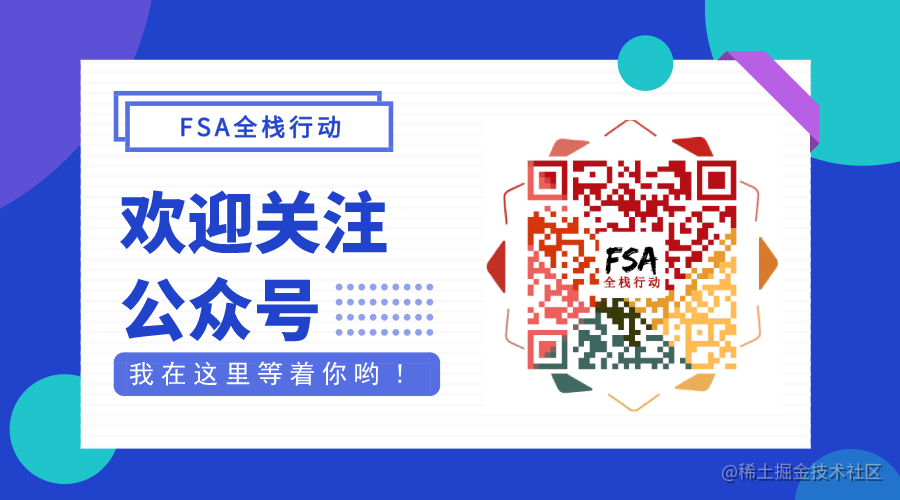# Kotlin - 数据类型

## Boolean 类型

``````val aBoolean: Boolean = true
val anotherBoolean: Boolean = false

kotlin 中的 Boolean 在多数情况下，相当于 Java 中基本类型 boolean，只有在必要情况下才会装箱成 Java 中的装箱类型 Boolean，由编译器决定，通常我们不需要关心。

## Number 类型

Number 即数字，主要包括整型和浮点型：

Float 32

Int 32
Short 16

### Int 类型

kotlin 的 Int 类型跟 Java 中的 int 一样，可以用整数、十六进制、二进制写法表示：

``````val anInt: Int = 8 // 8
val anotherInt: Int = 0xFF // 255
val moreInt: Int = 0b00000011 // 3
val maxInt: Int = Int.MAX_VALUE // 2147483647
val minInt: Int = Int.MIN_VALUE // -2147483648

### Long 类型

Long 类型与 Int 类型相似，只是 Int 类型是 32 位，而 Long 类型是 64 位，能表示更大的数：

``````val aLong: Long = 12345678987654321 // 12345678987654321
val anotherLong: Long = 123 // 123
val maxLong: Long = Long.MAX_VALUE // 9223372036854775807
val minLong: Long = Long.MIN_VALUE // -9223372036854775808

### Float 类型

Float 即单精度浮点数，是带有小数的数，Float 类型的数值表示必须在末尾加上 `F``f`，否则编译会认为是 Double 类型。

``````val aFloat: Float = 2.0F // 2.0
val anotherFloat: Float = 1E3f // 1000.0
val maxFloat: Float = Float.MAX_VALUE // 3.4028235E38
val minFloat: Float = Float.MIN_VALUE // 1.4E-45
val minFloatReal: Float = -Float.MAX_VALUE // -3.4028235E38
val positiveInfinityFloat: Float = Float.POSITIVE_INFINITY // 正无穷，输出 Infinity
val negativeInfinityFloat: Float = Float.NEGATIVE_INFINITY // 负无穷，输出 -Infinity

``````/**
* Represents a single-precision 32-bit IEEE 754 floating point number.
* On the JVM, non-nullable values of this type are represented as values of the primitive type `float`.
*/
public class Float private constructor() : Number(), Comparable<Float> {
companion object {
/**
* A constant holding the smallest *positive* nonzero value of Float.
*/
public val MIN_VALUE: Float

/**
* A constant holding the largest positive finite value of Float.
*/
public val MAX_VALUE: Float
}
}

#### NaN

Float 拥有一个 NaN 常量属性，表示"不是一个数"的数，什么情况下会得到 NaN 呢？

``````println(0.0f / 0.0f) // NaN
println(0.0f / 0.0f == Float.NaN) // false

1. 除零可以得到 NaN
2. NaN 跟任何 NaN 结果不是相等的

NaN 表示 "不是一个数" ，所以比较起来没有意义。

### Double 类型

Double 即双精度浮点数（或多精度浮点数），Double 之于 Float 就如同 Long 之 Int，故 Double 其实就是比 Float 能表示更大的浮点数而已。

``````val aDouble: Double = 3.0 // 3.0
val anotherDouble: Double = 3.1415926 // 3.1415926
val maxDouble: Double = Double.MAX_VALUE // 1.7976931348623157E308
val minDouble: Double = Double.MIN_VALUE // 4.9E-324
val minDoubleReal: Double = -Double.MAX_VALUE // -1.7976931348623157E308

Double 与 Float 一样，`Double.MIN_VALUE` 表示的是最小非 0 正数。

### Short 类型

Short 也称短整型，位宽比 Int 少一半，即 16 位：

``````val aShort: Short = 127 // 127
val maxShort: Short = Short.MAX_VALUE // 32767
val minShort: Short = Short.MIN_VALUE // -32768

### Byte 类型

Byte 类型比 Short 类型更短，一般只用来处理二进制数据流：

``````val maxByte: Byte = Byte.MAX_VALUE // 127
val minByte: Byte = Byte.MIN_VALUE // -128

### 基本类型转换

Java 支持使用 long 类型或 float 类型变量接收一个 int 类型变量，且不报错，这是因为 Java 支持隐式转换，但这在 Kotlin 中是行不通的，Kotlin 不支持隐式转换，举个例子：

``````val anInt: Int = 5
val aLong: Long = anInt // 报错：Type mismatch
val aLong: Long = anInt.toLong() // 正常

## Char 类型

• Char 称为字符，对应 Java 的 Character
• Char 占两个字节，表示一个 16 位的 Unicode 字符
• Char 需要用单引号 `''` 引起来，例如：'0'，'a'，'\n'
``````val aChar: Char = '0' // 0
val bChar: Char = '林' // 林
val cChar: Char = '\t' // 看不见的制表符
val dChar: Char = '\u9510' // 锐

Char 表示一个字符，其值的书写可以是字母、数字、Unicode、以及类似 `\n` 的特殊字符。

\t制表符
\b光标后退一个字符
\n回车
\r光标回到行首
\'单引号
\"双引号
\\反斜杠
\\$美元符号，Kotlin 支持美元符号开头的字符串模板
``````val eChar: Char = '\'' // '
val fChar: Char = '"' // "
val gChar: Char = '\"' // "
val hChar: Char = '\\$' // \$
val iChar: Char = '\\' // \

## String 类型

String 字符串，即一连串的字符，字符 Char 使用 `''`引起来，字符串 String 则使用 `""`引起来：

``````val aString: String = "HelloWorld" // HelloWorld
val anotherString: String = String(charArrayOf('H', 'e', 'l', 'l', 'o', 'W', 'o', 'r', 'l', 'd')) // HelloWorld
println(aString == anotherString) // true
println(aString === anotherString) // false
println(aString.length) // 10

``````println("输出一个字符串：" + aString) // HelloWorld
val arg1: Int = 0
val arg2: Int = 1
println("\$arg1 + \$arg2 = \${arg1 + arg2}") // 0 + 1 = 1

``````// 输出结果：
//    \t \n
//    |哈哈哈
val rawString: String = """
\t \n
|哈哈哈
"""
val rawString1: String = """\$aString  \\$aString""" // HelloWorld  \HelloWorld
val rawString2: String = """\$ aString""" // \$ aString